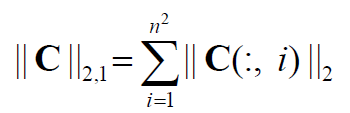# Could L21 norm be built in objective function?

Hello Everyone!
At present I am working with a project using Sparse Subspace coding technique. And I’m new to CVX.
it is only the l21 norm which locates in the g() function i cannot write into the objective function. The l21 norm is defined as this.

Could it be solved by cvx? Or other toolbox recommendations?

Presuming the sum is over all columns in C,
`sum(norms(C))`
should be what you want.

`````` help norms

norms   Computation of multiple vector norms.
norms( X ) provides a means to compute the norms of multiple vectors
packed into a matrix or N-D array. This is useful for performing
max-of-norms or sum-of-norms calculations.

All of the vector norms, including the false "-inf" norm, supported
by NORM() have been implemented in the norms() command.
norms(X,P)           = sum(abs(X).^P).^(1/P)
norms(X)             = norms(X,2).
norms(X,inf)         = max(abs(X)).
norms(X,-inf)        = min(abs(X)).
If X is a vector, these computations are completely identical to
their NORM equivalents. If X is a matrix, a row vector is returned
of the norms of each column of X. If X is an N-D matrix, the norms
are computed along the first non-singleton dimension.

norms( X, [], DIM ) or norms( X, 2, DIM ) computes Euclidean norms
along the dimension DIM. norms( X, P, DIM ) computes its norms
along the dimension DIM.

Disciplined convex programming information:
norms is convex, except when P<1, so an error will result if these
non-convex "norms" are used within CVX expressions. norms is
nonmonotonic, so its input must be affine.``````
2 Likes

Thanks a lot.
The example file uses a vector as variable. And from here I was taught that matrix could be variable and even as a parameter to a function used in the cvx sentence. Thank you for your help!